Home

# On the Size-Ramsey Number of Cycles

## Abstract

For given graphs G1,…, Gk, the size-Ramsey number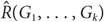$\hat R({G_1}, \ldots ,{G_k})$ is the smallest integer m for which there exists a graph H on m edges such that in every k-edge colouring of H with colours 1,…,k, H contains a monochromatic copy of Gi of colour i for some 1 ≤ ik. We denote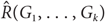$\hat R({G_1}, \ldots ,{G_k})$ by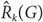${\hat R_k}(G)$ when G1 = ⋯ = Gk = G.

Haxell, Kohayakawa and Łuczak showed that the size-Ramsey number of a cycle Cn is linear in n,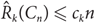${\hat R_k}({C_n}) \le {c_k}n$ for some constant ck. Their proof, however, is based on Szemerédi’s regularity lemma so no specific constant ck is known.

In this paper, we give various upper bounds for the size-Ramsey numbers of cycles. We provide an alternative proof of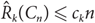${\hat R_k}({C_n}) \le {c_k}n$ , avoiding use of the regularity lemma, where ck is exponential and doubly exponential in k, when n is even and odd, respectively. In particular, we show that for sufficiently large n we have${\hat R_2}({C_n}) \le {10^5} \times cn$ , where c = 6.5 if n is even and c = 1989 otherwise.

## Footnotes

Hide All

Research partially supported by INSF grant no. 95844679.

Research partially carried out in the IPM-Isfahan Branch and in part supported by a grant from IPM (no. 95050217).

§

Research partially supported by SNSF grant 200021-149111.

Hide All

# On the Size-Ramsey Number of Cycles

## Metrics

### Full text viewsFull text views reflects the number of PDF downloads, PDFs sent to Google Drive, Dropbox and Kindle and HTML full text views.

Total number of HTML views: 0
Total number of PDF views: 0 *Loading metrics...

### Abstract viewsAbstract views reflect the number of visits to the article landing page.

Total abstract views: 0 *Loading metrics...

* Views captured on Cambridge Core between <date>. This data will be updated every 24 hours.

Usage data cannot currently be displayed